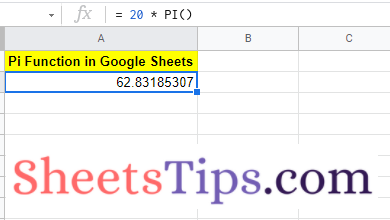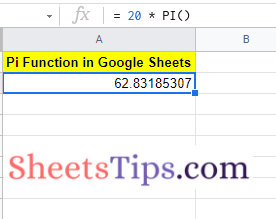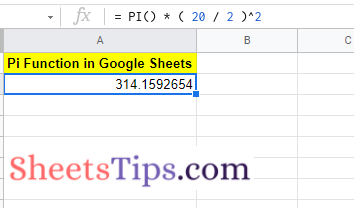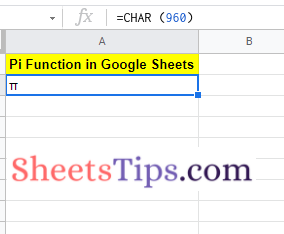# Pi Function in Google Sheets: Discover & Generate π Function in Google Sheets

Google Sheets allows users to even perform the most complex mathematical calculations. We can also use this software to perform Circle related calculations, such as finding circumference, diameter, area of a circle, and many others. However, to perform Circle related calculation, we will have to use the Pi (π) functions. Pi (π) is one of the most important mathematical operations which is widely used in Circle related calculations. On this page, we will understand how to use the Pi function to find the Circle related calculations using Google Sheets Tips. Read further to find more.## Pi Function for Circle Calculations – Syntax

To enable the Pi function in Google Sheets, we will have to use the syntax. The syntax to use the Pi function in Google Sheet is as follows:

=PI()

It is to be noted that there are no arguments in between the brackets. If you use any arguments in the bracket, then the Pi function will return an error.

### Using Pi Functions in Mathematical Calculations

In order to perform mathematical calculations, you will have to know the circumference of the circle. If you know the circumference of the circle, then you can easily calculate the diameter and other calculations of the circle.

### Calculating Circle Circumference in Google Sheets using Pi Function

To calculate the circumference of the circle in Google Sheets, you will have to know the diameter of the circle. Let us assume that you want to find the circumference of the circle, whose diameter value is 20 cm. The steps to find the circumference is as follows:

• 1st Step: Open the Google Sheets where you want to calculate the circumference of the circle.
• 2nd Step: Move to the cell where you want to draw the results.
• 3rd Step: Now simply enter the formula = 20 * PI().
• 4th Step: Press the Return Key and you will see the results as shown below. The value returned is the circumference of the circle.### Calculating Circle Diameter in Google Sheets using Pi Function

To evaluate the diameter of the circle, we will have to know the circumference value. Suppose if your circumference value of the circle is 20cm, then you simply follow the steps outlined below to know the diameter of the circle.

• 2nd Step: Now move to the cell where you want to find the diameter value of a circle.
• 3rd Step: Now use the formula =20*PI().
• 4th Step: Press the “Enter” button and you will see the results as shown in the below image.### Calculating Area of Circle in Google Sheets using Pi Function

Google Sheets also allows the user to calculate the area of the circle using the Pi function. To calculate the area of the circle using the Pi function, we will have to first know the area of the circle formula. The area of the circle formula is π * r².

Thus we have to know the radius value. To find the radius of the circle, we will have to divide the diameter by 2. In the previous sections, we have learned how to find the diameter of the circle. Use those formulas to find the diameter of the circle.

Now let us assume that the diameter value of the circle is 20cm. The steps to find the area of a circle in Google Sheets using this data is as follows:

• 2nd Step: Now move to the cell where you want to find the area of the circle.
• 3rd Step: Enter the formula = PI() * ( 20 / 2 )^2.
• 4th Step: Press the Return key and you will find the results as shown below.### How to Make a PI Symbol in Google Sheets?

We can easily generate the PI symbol in a Google Spreadsheet using the CHAR function. Follow the steps as listed below to generate the PI symbol in Google Sheets:

• 1st Step: Open Google Sheets on your device where you want to generate the PI symbol.
• 2nd Step: Move to the cell where you want to generate the PI symbol.
• 3rd Step: Enter the character function formula as =CHAR (960).
• 4th Step: Press the “Enter” key and you will see the results as shown below. Here you will see the PI symbol being generated in Google Sheets.Fun Fact: Google Sheets also lets users generate different pies. Want to know how to do this. Well, follow the steps outlined below:

• 1st Step: Move to the cell where you want to generate the pie in Google Sheets.
• 2nd Step: Simply enter the formula =CHAR(129383).
• 3rd Step: Press the return key and you will see the results.